# IfExtend.au3 - include file for enabling shorter scripts

## Recommended Posts

Although my documentation of these functions is not very good, I feel that others may benefit from this include. I always enjoyed coding in Javascript because I could do single-line if-then-else statements. I also always wanted a way to compare a single variable with multiple values. Ternary operations have brought me closer to this goal, but there is yet a distance to go. These functions should help others to make shorter code.

IfExtend.au3

```#include-once

; IfThenElse - A one-line If Then Else EndIf statement for code shortening. It allows for one function with parameters if true, one function with parameters if false
;
; IfThenElse(expression to test, value to test against, Function 1, [Function 1 parameters wrapped with param(), [Function 2, [Function 2 parameters wrapped with param()]]])
;
; Usage example:
; IfThenElse(\$sky = "falling", MsgBox, param(0, "", "Sky is falling"), MsgBox, param(0, "", "Sky is not falling"))
;
; param(..., ..., ...) is for a list of parameters to be passed to the functions.

Func IfThenElse(\$test, \$func1, \$params1 = Null, \$func2 = Null, \$params2 = Null)
If \$test Then
If \$params1 = Null Then
Return \$func1()
Else
Return Call(FuncName(\$func1), \$params1)
EndIf
Else
If \$func2 = Null Then Return -1
If \$params2 = Null Then
Return \$func2()
Else
Return Call(FuncName(\$func2), \$params2)
EndIf
EndIf
EndFunc

Func param(\$1=0, \$2=0, \$3=0, \$4=0, \$5=0, \$6=0, \$7=0, \$8=0, \$9=0, \$10=0, \$11=0, \$12=0, \$13=0, \$14=0, \$15=0, \$16=0, \$17=0, \$18=0, \$19=0, \$20=0, \$21=0, \$22=0, \$23=0, \$24=0, \$25=0, \$26=0, \$27=0, \$28=0, \$29=0, \$30=0, \$31=0, \$32=0, \$33=0, \$34=0, \$35=0, \$36=0, \$37=0, \$38=0, \$39=0, \$40=0, \$41=0, \$42=0, \$43=0, \$44=0, \$45=0, \$46=0, \$47=0, \$48=0, \$49=0, \$50=0, \$51=0, \$52=0, \$53=0, \$54=0, \$55=0, \$56=0, \$57=0, \$58=0, \$59=0, \$60=0, \$61=0, \$62=0, \$63=0, \$64=0, \$65=0, \$66=0, \$67=0, \$68=0, \$69=0, \$70=0, \$71=0, \$72=0, \$73=0, \$74=0, \$75=0, \$76=0, \$77=0, \$78=0, \$79=0, \$80=0, \$81=0, \$82=0, \$83=0, \$84=0, \$85=0, \$86=0, \$87=0, \$88=0, \$89=0, \$90=0, \$91=0, \$92=0, \$93=0, \$94=0, \$95=0, \$96=0, \$97=0, \$98=0, \$99=0, \$100=0, \$101=0, \$102=0, \$103=0, \$104=0, \$105=0, \$106=0, \$107=0, \$108=0, \$109=0, \$110=0, \$111=0, \$112=0, \$113=0, \$114=0, \$115=0, \$116=0, \$117=0, \$118=0, \$119=0, \$120=0, \$121=0, \$122=0, \$123=0, \$124=0, \$125=0, \$126=0, \$127=0, \$128=0, \$129=0, \$130=0, \$131=0, \$132=0, \$133=0, \$134=0, \$135=0, \$136=0, \$137=0, \$138=0, \$139=0, \$140=0, \$141=0, \$142=0, \$143=0, \$144=0, \$145=0, \$146=0, \$147=0, \$148=0, \$149=0, \$150=0, \$151=0, \$152=0, \$153=0, \$154=0, \$155=0, \$156=0, \$157=0, \$158=0, \$159=0, \$160=0, \$161=0, \$162=0, \$163=0, \$164=0, \$165=0, \$166=0, \$167=0, \$168=0, \$169=0, \$170=0, \$171=0, \$172=0, \$173=0, \$174=0, \$175=0, \$176=0, \$177=0, \$178=0, \$179=0, \$180=0, \$181=0, \$182=0, \$183=0, \$184=0, \$185=0, \$186=0, \$187=0, \$188=0, \$189=0, \$190=0, \$191=0, \$192=0, \$193=0, \$194=0, \$195=0, \$196=0, \$197=0, \$198=0, \$199=0, \$200=0, \$201=0, \$202=0, \$203=0, \$204=0, \$205=0, \$206=0, \$207=0, \$208=0, \$209=0, \$210=0, \$211=0, \$212=0, \$213=0, \$214=0, \$215=0, \$216=0, \$217=0, \$218=0, \$219=0, \$220=0, \$221=0, \$222=0, \$223=0, \$224=0, \$225=0, \$226=0, \$227=0, \$228=0, \$229=0, \$230=0, \$231=0, \$232=0, \$233=0, \$234=0, \$235=0, \$236=0, \$237=0, \$238=0, \$239=0, \$240=0, \$241=0, \$242=0, \$243=0, \$244=0, \$245=0, \$246=0, \$247=0, \$248=0, \$249=0, \$250=0, \$251=0, \$252=0, \$253=0, \$254=0, \$255=0)
Local \$arr[@NumParams + 1] = ["CallArgArray"]
For \$i = 1 To @NumParams
\$arr[\$i] = Eval(\$i)
Next
Return \$arr
EndFunc

Func MultiOr(\$value, \$2=0, \$3=0, \$4=0, \$5=0, \$6=0, \$7=0, \$8=0, \$9=0, \$10=0, \$11=0, \$12=0, \$13=0, \$14=0, \$15=0, \$16=0, \$17=0, \$18=0, \$19=0, \$20=0, \$21=0, \$22=0, \$23=0, \$24=0, \$25=0, \$26=0, \$27=0, \$28=0, \$29=0, \$30=0, \$31=0, \$32=0, \$33=0, \$34=0, \$35=0, \$36=0, \$37=0, \$38=0, \$39=0, \$40=0, \$41=0, \$42=0, \$43=0, \$44=0, \$45=0, \$46=0, \$47=0, \$48=0, \$49=0, \$50=0, \$51=0, \$52=0, \$53=0, \$54=0, \$55=0, \$56=0, \$57=0, \$58=0, \$59=0, \$60=0, \$61=0, \$62=0, \$63=0, \$64=0, \$65=0, \$66=0, \$67=0, \$68=0, \$69=0, \$70=0, \$71=0, \$72=0, \$73=0, \$74=0, \$75=0, \$76=0, \$77=0, \$78=0, \$79=0, \$80=0, \$81=0, \$82=0, \$83=0, \$84=0, \$85=0, \$86=0, \$87=0, \$88=0, \$89=0, \$90=0, \$91=0, \$92=0, \$93=0, \$94=0, \$95=0, \$96=0, \$97=0, \$98=0, \$99=0, \$100=0, \$101=0, \$102=0, \$103=0, \$104=0, \$105=0, \$106=0, \$107=0, \$108=0, \$109=0, \$110=0, \$111=0, \$112=0, \$113=0, \$114=0, \$115=0, \$116=0, \$117=0, \$118=0, \$119=0, \$120=0, \$121=0, \$122=0, \$123=0, \$124=0, \$125=0, \$126=0, \$127=0, \$128=0, \$129=0, \$130=0, \$131=0, \$132=0, \$133=0, \$134=0, \$135=0, \$136=0, \$137=0, \$138=0, \$139=0, \$140=0, \$141=0, \$142=0, \$143=0, \$144=0, \$145=0, \$146=0, \$147=0, \$148=0, \$149=0, \$150=0, \$151=0, \$152=0, \$153=0, \$154=0, \$155=0, \$156=0, \$157=0, \$158=0, \$159=0, \$160=0, \$161=0, \$162=0, \$163=0, \$164=0, \$165=0, \$166=0, \$167=0, \$168=0, \$169=0, \$170=0, \$171=0, \$172=0, \$173=0, \$174=0, \$175=0, \$176=0, \$177=0, \$178=0, \$179=0, \$180=0, \$181=0, \$182=0, \$183=0, \$184=0, \$185=0, \$186=0, \$187=0, \$188=0, \$189=0, \$190=0, \$191=0, \$192=0, \$193=0, \$194=0, \$195=0, \$196=0, \$197=0, \$198=0, \$199=0, \$200=0, \$201=0, \$202=0, \$203=0, \$204=0, \$205=0, \$206=0, \$207=0, \$208=0, \$209=0, \$210=0, \$211=0, \$212=0, \$213=0, \$214=0, \$215=0, \$216=0, \$217=0, \$218=0, \$219=0, \$220=0, \$221=0, \$222=0, \$223=0, \$224=0, \$225=0, \$226=0, \$227=0, \$228=0, \$229=0, \$230=0, \$231=0, \$232=0, \$233=0, \$234=0, \$235=0, \$236=0, \$237=0, \$238=0, \$239=0, \$240=0, \$241=0, \$242=0, \$243=0, \$244=0, \$245=0, \$246=0, \$247=0, \$248=0, \$249=0, \$250=0, \$251=0, \$252=0, \$253=0, \$254=0, \$255=0)
For \$i = 2 to @NumParams
If \$value = Eval(\$i) Then Return 1
Next
Return 0
EndFunc

Func MultiNot(\$value, \$2=0, \$3=0, \$4=0, \$5=0, \$6=0, \$7=0, \$8=0, \$9=0, \$10=0, \$11=0, \$12=0, \$13=0, \$14=0, \$15=0, \$16=0, \$17=0, \$18=0, \$19=0, \$20=0, \$21=0, \$22=0, \$23=0, \$24=0, \$25=0, \$26=0, \$27=0, \$28=0, \$29=0, \$30=0, \$31=0, \$32=0, \$33=0, \$34=0, \$35=0, \$36=0, \$37=0, \$38=0, \$39=0, \$40=0, \$41=0, \$42=0, \$43=0, \$44=0, \$45=0, \$46=0, \$47=0, \$48=0, \$49=0, \$50=0, \$51=0, \$52=0, \$53=0, \$54=0, \$55=0, \$56=0, \$57=0, \$58=0, \$59=0, \$60=0, \$61=0, \$62=0, \$63=0, \$64=0, \$65=0, \$66=0, \$67=0, \$68=0, \$69=0, \$70=0, \$71=0, \$72=0, \$73=0, \$74=0, \$75=0, \$76=0, \$77=0, \$78=0, \$79=0, \$80=0, \$81=0, \$82=0, \$83=0, \$84=0, \$85=0, \$86=0, \$87=0, \$88=0, \$89=0, \$90=0, \$91=0, \$92=0, \$93=0, \$94=0, \$95=0, \$96=0, \$97=0, \$98=0, \$99=0, \$100=0, \$101=0, \$102=0, \$103=0, \$104=0, \$105=0, \$106=0, \$107=0, \$108=0, \$109=0, \$110=0, \$111=0, \$112=0, \$113=0, \$114=0, \$115=0, \$116=0, \$117=0, \$118=0, \$119=0, \$120=0, \$121=0, \$122=0, \$123=0, \$124=0, \$125=0, \$126=0, \$127=0, \$128=0, \$129=0, \$130=0, \$131=0, \$132=0, \$133=0, \$134=0, \$135=0, \$136=0, \$137=0, \$138=0, \$139=0, \$140=0, \$141=0, \$142=0, \$143=0, \$144=0, \$145=0, \$146=0, \$147=0, \$148=0, \$149=0, \$150=0, \$151=0, \$152=0, \$153=0, \$154=0, \$155=0, \$156=0, \$157=0, \$158=0, \$159=0, \$160=0, \$161=0, \$162=0, \$163=0, \$164=0, \$165=0, \$166=0, \$167=0, \$168=0, \$169=0, \$170=0, \$171=0, \$172=0, \$173=0, \$174=0, \$175=0, \$176=0, \$177=0, \$178=0, \$179=0, \$180=0, \$181=0, \$182=0, \$183=0, \$184=0, \$185=0, \$186=0, \$187=0, \$188=0, \$189=0, \$190=0, \$191=0, \$192=0, \$193=0, \$194=0, \$195=0, \$196=0, \$197=0, \$198=0, \$199=0, \$200=0, \$201=0, \$202=0, \$203=0, \$204=0, \$205=0, \$206=0, \$207=0, \$208=0, \$209=0, \$210=0, \$211=0, \$212=0, \$213=0, \$214=0, \$215=0, \$216=0, \$217=0, \$218=0, \$219=0, \$220=0, \$221=0, \$222=0, \$223=0, \$224=0, \$225=0, \$226=0, \$227=0, \$228=0, \$229=0, \$230=0, \$231=0, \$232=0, \$233=0, \$234=0, \$235=0, \$236=0, \$237=0, \$238=0, \$239=0, \$240=0, \$241=0, \$242=0, \$243=0, \$244=0, \$245=0, \$246=0, \$247=0, \$248=0, \$249=0, \$250=0, \$251=0, \$252=0, \$253=0, \$254=0, \$255=0)
For \$i = 2 to @NumParams
If \$value = Eval(\$i) Then Return 0
Next
Return 1
EndFunc```

IfExtend_example.au3

```#include "IfExtend.au3"

\$sky = InputBox("Sky status", "Play around with values like blue, falling, green.", "falling")

#cs
;The following code can now be expressed as one-liners:

If \$sky = "falling" Then
MsgBox(0, "Test 1", "Sky is falling")
Else
MsgBox(0, "Test 1", "Sky is not falling")
EndIf

If \$sky = "blue" Or \$sky = "falling" Then
MsgBox(0, "Test 2", "Sky is blue or falling")
Else
MsgBox(0, "Test 2", "Sky is not blue or falling")
EndIf

If \$sky <> "blue" And \$sky <> "falling" Then
MsgBox(0, "Test 3", "Sky is not blue or falling")
Else
MsgBox(0, "Test 3", "Sky is blue or falling")
EndIf
#ce

IfThenElse(\$sky = "falling", MsgBox, param(0, "Test 1", "Sky is falling"), MsgBox, param(0, "Test 1", "Sky is not falling"))
IfThenElse(MultiOr(\$sky, "blue", "falling"), MsgBox, param(0, "Test 2", "Sky is blue or falling"), MsgBox, param(0, "Test 2", "Sky is not blue or falling"))
IfThenElse(MultiNot(\$sky, "blue", "falling"), MsgBox, param(0, "Test 3", "Sky is not blue or falling"), MsgBox, param(0, "Test 3", "Sky is blue or falling"))

; MultiNot Example
;
; Old code:
; If \$sky <> "blue" And \$sky <> "falling" And \$sky <> "red" And \$sky <> "orange" And \$sky <> "green" Then
;
; New code:
; If MultiNot(\$sky, "blue", "falling", "red", "orange", "green") Then```

The entire purpose of these functions is to make shorter code. I hope they will be useful to others as well.

Who else would I be?

##### Share on other sites

Just keep it simple: (AutoIt 3.3.10.0+)

```\$sky = InputBox("Sky status", "Play around with values like blue, falling, green.", "falling")
MsgBox(0, "Test 1", "Sky is " & (\$sky = "falling" ? "" : "not ") & "falling")
MsgBox(0, "Test 2", "Sky is " & ((\$sky = "blue" Or \$sky = "falling") ? "" : "not ") & "blue or falling")
MsgBox(0, "Test 3", "Sky is " & ((\$sky <> "blue" And \$sky <> "falling") ? "not " : "") & "blue or falling")```
Edited by Exit

App: Au3toCmd              UDF: _SingleScript()

##### Share on other sites

Although that is a better example of the EXACT example I gave, there are other situations where one needs to call one function if true, another if false. The example I gave was not perfect in that respect. However, it was only an example.

Who else would I be?

##### Share on other sites

Just keep it simple: (AutoIt 3.3.10.1+)

```\$sky = InputBox("Sky status", "Play around with values like blue, falling, green.", "falling")
MsgBox(0, "Test 1", "Sky is " & (\$sky = "falling" ? "" : "not ") & "falling")
MsgBox(0, "Test 2", "Sky is " & ((\$sky = "blue" Or \$sky = "falling") ? "" : "not ") & "blue or falling")
MsgBox(0, "Test 3", "Sky is " & ((\$sky <> "blue" And \$sky <> "falling") ? "not " : "") & "blue or falling")```

This works in v3.3.10.0+

##### Share on other sites

Although that is a better example of the EXACT example I gave, there are other situations where one needs to call one function if true, another if false. The example I gave was not perfect in that respect. However, it was only an example.

Please give an example of other situations, where your UDF creates shorter one-liners than normal coding.

App: Au3toCmd              UDF: _SingleScript()

##### Share on other sites

Simple.

`IfThenElse(\$sky = "falling", MsgBox, param(0, "WARNING", "WARNING: This is important! The sky is falling!!!"), ConsoleWrite, param("It's a normal day." & @CRLF))`

Is one line where

```If \$sky = "falling" Then
MsgBox(0, "WARNING", "WARNING: This is important! The sky is falling!!!")
Else
ConsoleWrite("It's a normal day." & @CRLF)
EndIf```

Is five lines.

The whole point is to create a single line where two functions can be executed, based on an if statement.

The shortest possible way to code this without so many lines and without my functions that I know of is this:

```If \$sky = "falling" Then MsgBox(0, "WARNING", "WARNING: This is important! The sky is falling!!!")
If \$sky <> "falling" Then ConsoleWrite("It's a normal day." & @CRLF)```

Which is still two lines instead of one.

Edited by this-is-me

Who else would I be?

##### Share on other sites

How about like this using the 3.3.10+ syntax

```\$sky = InputBox("Sky status", "Play around with values like blue, falling, green.", "green")
(\$sky = "falling") ? MsgBox(0, "test1", "sky is falling") : ConsoleWrite("It's a normal day." & @CRLF)```

If I posted any code, assume that code was written using the latest release version unless stated otherwise. Also, if it doesn't work on XP I can't help with that because I don't have access to XP, and I'm not going to.
Give a programmer the correct code and he can do his work for a day. Teach a programmer to debug and he can do his work for a lifetime - by Chirag Gude
How to ask questions the smart way!

I hereby grant any person the right to use any code I post, that I am the original author of, on the autoitscript.com forums, unless I've specifically stated otherwise in the code or the thread post. If you do use my code all I ask, as a courtesy, is to make note of where you got it from.

Back up and restore Windows user files _Array.au3 - Modified array functions that include support for 2D arrays.  -  ColorChooser - An add-on for SciTE that pops up a color dialog so you can select and paste a color code into a script.  -  Customizable Splashscreen GUI w/Progress Bar - Create a custom "splash screen" GUI with a progress bar and custom label.  -  _FileGetProperty - Retrieve the properties of a file  -  SciTE Toolbar - A toolbar demo for use with the SciTE editor  -  GUIRegisterMsg demo - Demo script to show how to use the Windows messages to interact with controls and your GUI.  -   Latin Square password generator

##### Share on other sites

@BrewManNH,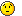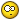That didn't work for me when I tried it before! I probably had the syntax wrong. That's totally and completely what I wanted. I have no idea why it didn't work...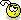Yet, I am so very happy that it works!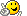EDIT: if you have a suggestion for replacing MultiOr and MultiNot with some built-in functions I am happy to listen.Edited by this-is-me

Who else would I be?

##### Share on other sites

MultiOr?

```Switch \$var
Case \$val1, \$val2, \$val3, \$val4
; do something
Case Else
; \$var is not = any \$vali
EndSwitch```

This wonderful site allows debugging and testing regular expressions (many flavors available). An absolute must have in your bookmarks.
RegExp tutorial: enough to get started
PCRE v8.33 regexp documentation latest available release and currently implemented in AutoIt beta.

SQLitespeed is another feature-rich premier SQLite manager (includes import/export). Well worth a try.
SQLite Expert (freeware Personal Edition or payware Pro version) is a very useful SQLite database manager.
An excellent eBook covering almost every aspect of SQLite3: a must-read for anyone doing serious work.
SQL tutorial (covers "generic" SQL, but most of it applies to SQLite as well)
A work-in-progress SQLite3 tutorial. Don't miss other LxyzTHW pages!
SQLite official website with full documentation (may be newer than the SQLite library that comes standard with AutoIt)

##### Share on other sites

@jchd, Thanks for your example, it's one that others might benefit from, even though it might not be a good replacement for MultiOr. I am specifically looking for examples that allow a code to be fewer lines.

Who else would I be?

##### Share on other sites

this-is-me,

I am specifically looking for examples that allow a code to be fewer lines

If I may - that is not something that should most definitely not, in my opinion, drive a coding process. I would put reliability and maintainability much, much higher up the list of requirements than pure size. As an interpreted language with a built-in interpreter, AutoIt does not produce the smallest executables around, so trying to save one or two lines of codes by using complex and difficult to understand functions and structures is not going to make a great deal of difference to the final size of the compiled script. Much better to make sure that the code is reliable (by using additional error-checking) and maintainable (by using simple code structures and sensible variable names) so that when you return to the code in a few months (or weeks or days depending on your age) you can easily work out what is going on and where and how to modify the code to meet the changed circumstances it is now required to face.This thread, although arguing against "fastest speed" rather that "smallest size", might prove interesting reading as many of the comments could be directly applied to the other case.M23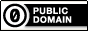Any of my own code posted anywhere on the forum is available for use by others without any restriction of any kind

Open spoiler to see my UDFs:

Spoiler

ArrayMultiColSort ---- Sort arrays on multiple columns
ChooseFileFolder ---- Single and multiple selections from specified path treeview listing
Date_Time_Convert -- Easily convert date/time formats, including the language used
ExtMsgBox --------- A highly customisable replacement for MsgBox
GUIExtender -------- Extend and retract multiple sections within a GUI
GUIFrame ---------- Subdivide GUIs into many adjustable frames
GUIListViewEx ------- Insert, delete, move, drag, sort, edit and colour ListView items
GUITreeViewEx ------ Check/clear parent and child checkboxes in a TreeView
Marquee ----------- Scrolling tickertape GUIs
NoFocusLines ------- Remove the dotted focus lines from buttons, sliders, radios and checkboxes
Notify ------------- Small notifications on the edge of the display
Scrollbars ----------Automatically sized scrollbars with a single command
StringSize ---------- Automatically size controls to fit text
Toast -------------- Small GUIs which pop out of the notification area

##### Share on other sites

Melba, I understand your point of view. In my case, it's a readability issue, instead of a file size issue. Readability (and comprehension) improves for me with wide code. My screen is a standard 16:9 wide screen 1080p resolution. Because of this, there is an ample amount of line width in the viewport, but a (relatively) small amount of height for coding (60 lines normally). If I were able to stand my monitor on its side, or have SciTE show a dual-pane reading view (where the right column of code would scroll simultaneously starting at the bottom of the left column), the problem would be solved. Writing code with width in mind instead of height allows me to comprehend code better by giving me a larger scope of the code in my view. This is one reason I don't (generally) use line continuation, or the standard 80-characters-wide coding style.

Now, bear in mind that I don't discount your recommendation. I am simply mentioning something that helps me in the long run. However, now that I think about it, I might be able to come up with a dual-pane SciTE editor and manage to fix this so-called "problem" I have once and for all. Then I won't be worried about overly-long code vs wide code. That might be my next project.

Thank you for your opinion. I do, indeed, have the problem that I have no idea what my code is doing after a few months, due to the fact that most of the time, I am learning what I am coding as I am coding it, and therefore only have a superficial understanding of how it works. That combined with the fact that I am often guilty of not commenting every part of my code makes for some interesting reading later.Who else would I be?

## Create an account

Register a new account

• ### Recently Browsing   0 members

×

• Wiki

• Back

• #### Beta

• Git
• FAQ
• Our Picks
×
• Create New...